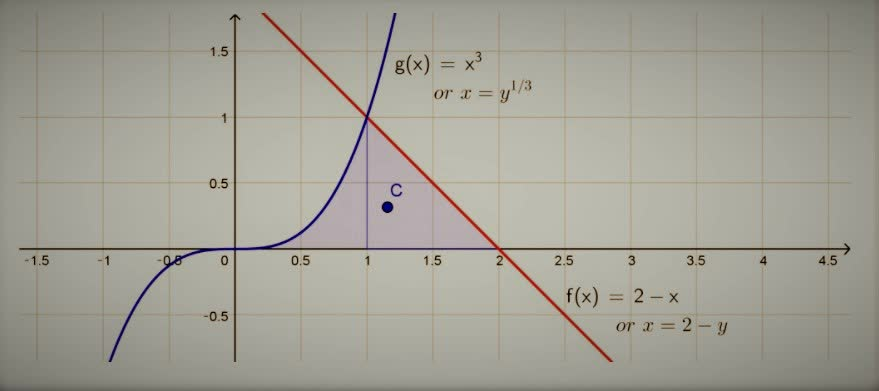# Find the centroid of the region bounded by the given curves. y=x^{3},x+y=2,y=Concepcion Hale 2021-12-10 Answered
Find the centroid of the region bounded by the given curves. $y={x}^{3},x+y=2,y=0$
You can still ask an expert for help

• Questions are typically answered in as fast as 30 minutes

Solve your problem for the price of one coffee

• Math expert for every subject
• Pay only if we can solve itLinda Birchfield
$f\left(x\right)=2-x$, $g\left(x\right)={x}^{3}$
or $x=2-y$, $x={y}^{\frac{1}{3}}$
They intersect at (1,1)
Since integrating with respect to x would mean we need to do two separate integrals for everything (from $x=0$ to l and from $x=1$ to 2), we could alternatively integrate with respect to y, where $x=2$ — y is the "top" function and $x={y}^{\frac{1}{3}}$ is the "bottom",Find the area of the bounded region.
$A={\int }_{0}^{1}\left(\left(2-y\right)-{y}^{\frac{1}{3}}\right)dy$
$={\left[2y-\frac{1}{2}{y}^{2}-\frac{3}{4}{y}^{\frac{4}{3}}\right]}_{0}^{1}$
$=2-\frac{1}{2}-\frac{3}{4}-\left(0-0-0\right)$
$=\frac{8-2-3}{4}$
$=\frac{3}{4}$
If we change all the x to y in the formula to find $\stackrel{―}{x}$, it will give the y coordinate of the centroid.
$\stackrel{―}{y}=\frac{1}{\frac{3}{4}}{\int }_{0}^{1}y\left(\left(2-y\right)-{y}^{\frac{1}{3}}\right)dy=\frac{4}{3}{\int }_{0}^{1}\left(2y-{y}^{2}-{y}^{\frac{4}{3}}\right)dy$
$=\frac{4}{3}{\left[{y}^{2}-\frac{1}{3}{y}^{3}-\frac{3}{7}{y}^{\frac{7}{3}}\right]}_{0}^{1}$
$=\frac{4}{3}\left[1-\frac{1}{3}-\frac{3}{7}-\left(0-0-0\right)\right]$
$=\frac{4}{3}\left[\frac{21-7-9}{21}\right]$
$=\frac{4}{3}\left[\frac{5}{21}\right]$
$=\frac{20}{63}$
Likewise, if we change all the x to y in the formula to find $\stackrel{―}{y}$, it will give the x coordinate of the centroid.lalilulelo2k3eq
Say f(x) and g(x) are the two bounding functions over $\left[a,b\right]$
The mass is $M={\int }_{a}^{b}f\left(x\right)-g\left(x\right)dx$
We find the moments:
${M}_{x}=\frac{1}{2}{\int }_{a}^{b}\left({\left[f\left(x\right)\right]}^{2}-{\left[g\left(x\right)\right]}^{2}\right)dx$
${M}_{y}={\int }_{a}^{b}x\left(f\left(x\right)-g\left(x\right)\right)dx$
And the center of mass, $\left(\stackrel{―}{x},\stackrel{―}{y}\right)$, is
$\stackrel{―}{x}=\frac{{M}_{y}}{M}$
$\stackrel{―}{y}=\frac{{M}_{x}}{M}$